# LEC 12-DSALGO-GRAPHS(final12).pdf

7. Apr 2022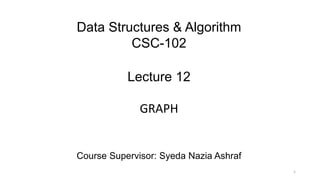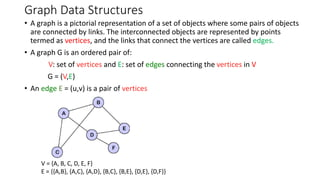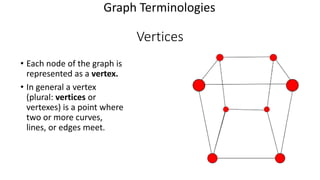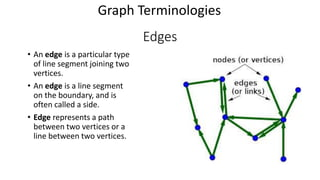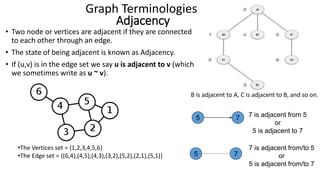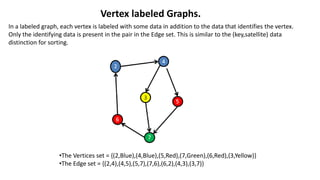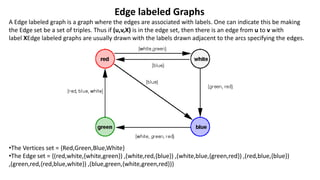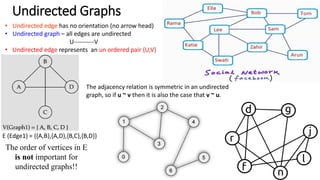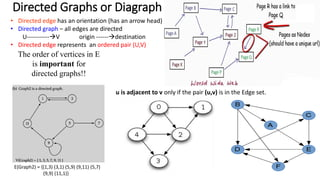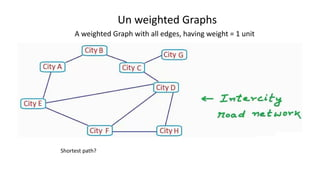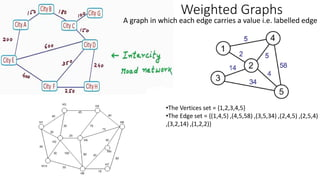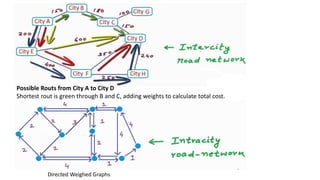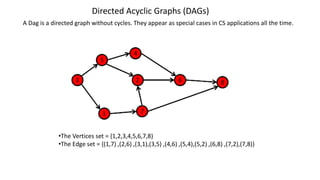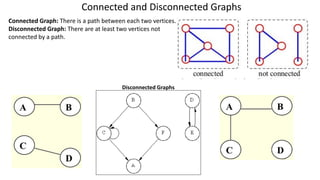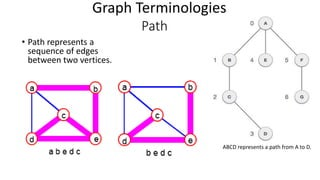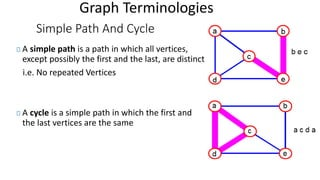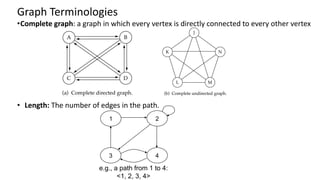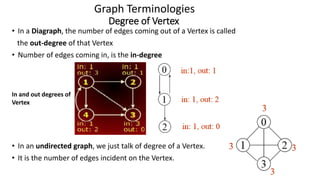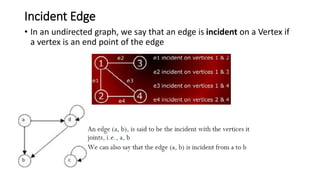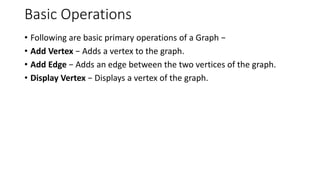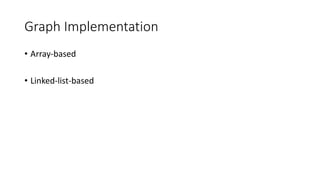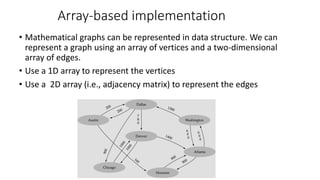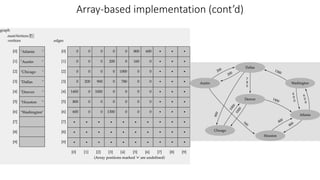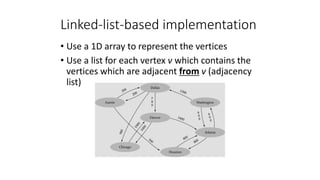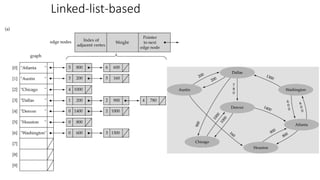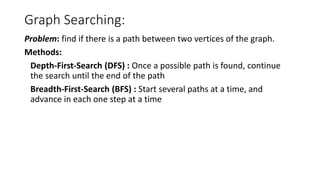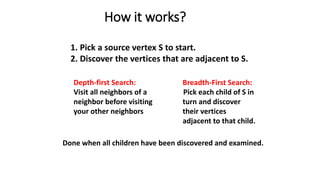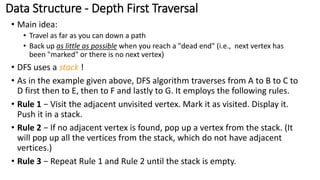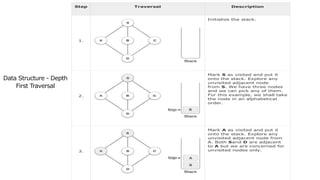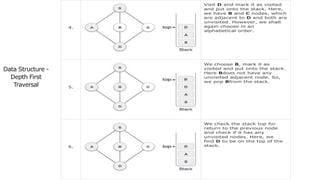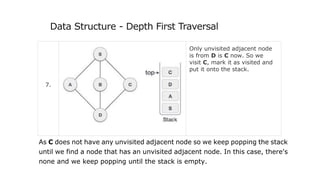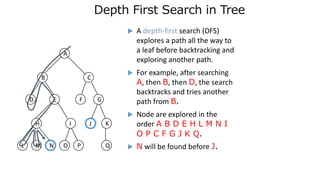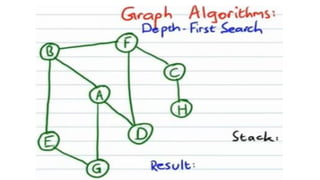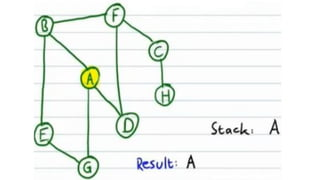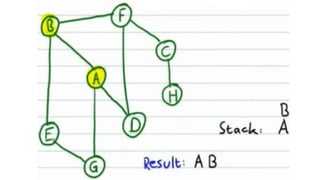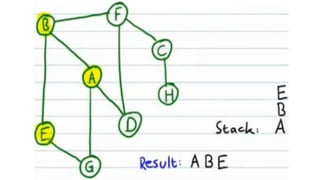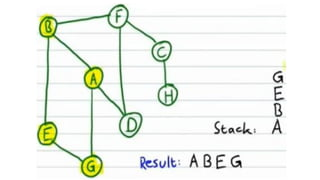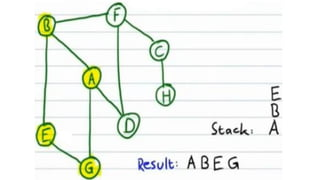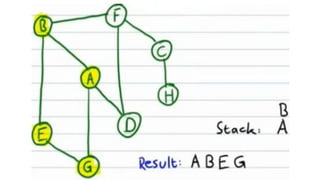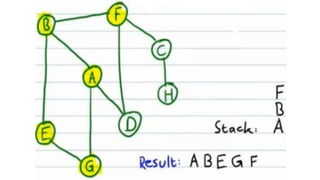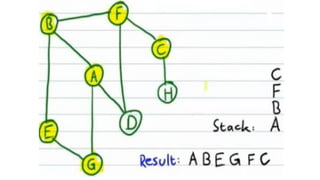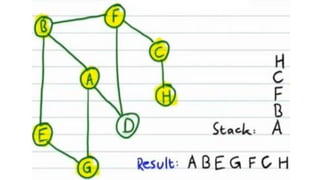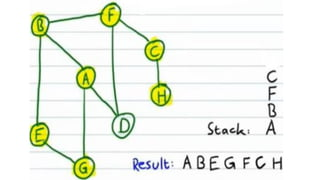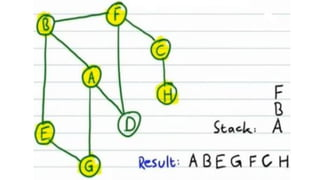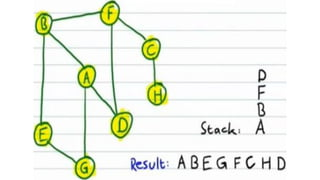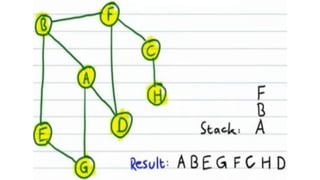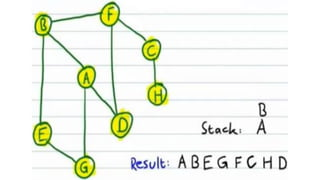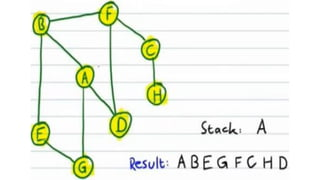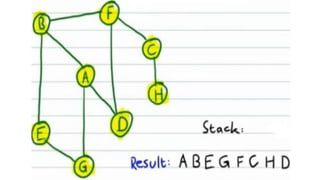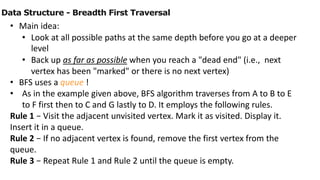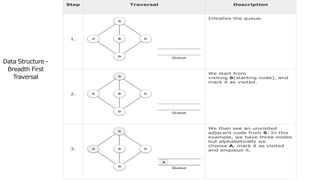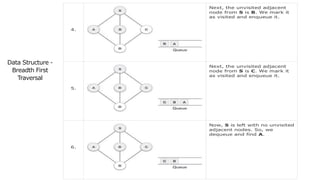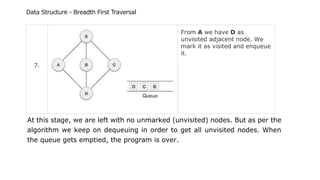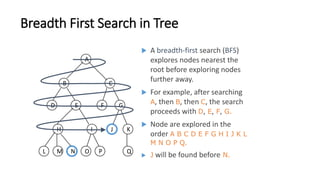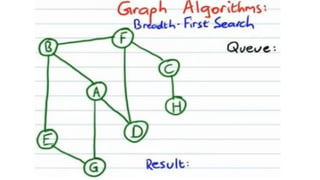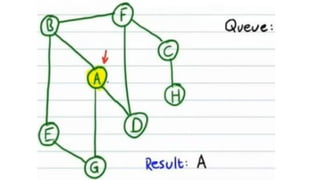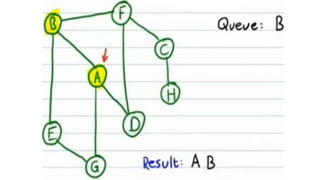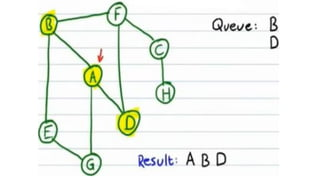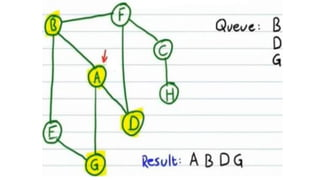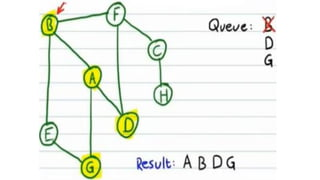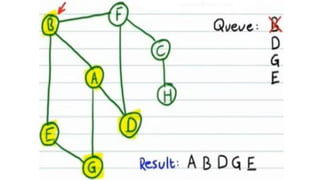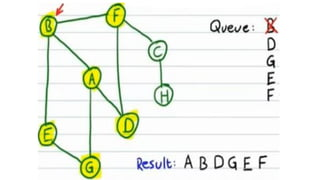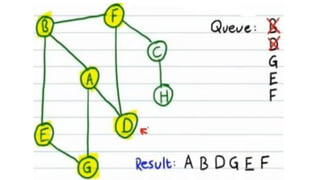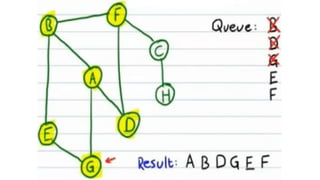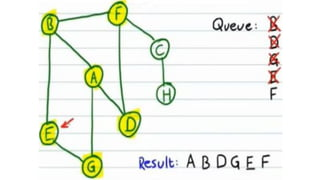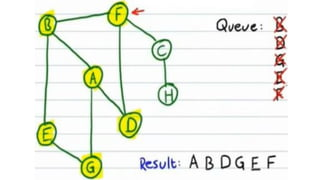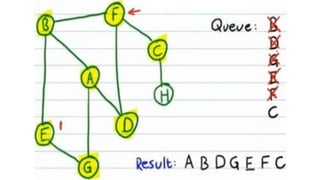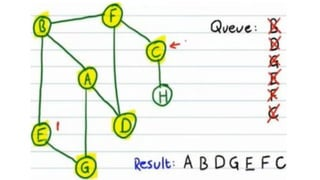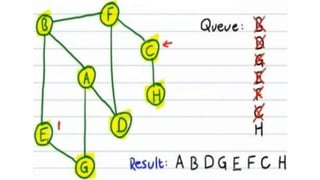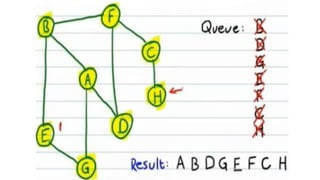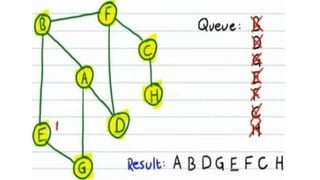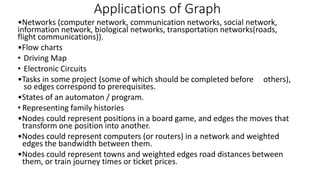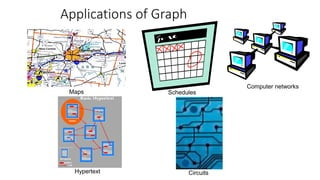1 von 73

### LEC 12-DSALGO-GRAPHS(final12).pdf

• 1. Data Structures & Algorithm CSC-102 Lecture 12 GRAPH Course Supervisor: Syeda Nazia Ashraf 1
• 2. Graph Data Structures • A graph is a pictorial representation of a set of objects where some pairs of objects are connected by links. The interconnected objects are represented by points termed as vertices, and the links that connect the vertices are called edges. • A graph G is an ordered pair of: V: set of vertices and E: set of edges connecting the vertices in V G = (V,E) • An edge E = (u,v) is a pair of vertices V = {A, B, C, D, E, F} E = {{A,B}, {A,C}, {A,D}, {B,C}, {B,E}, {D,E}, {D,F}}
• 3. Vertices • Each node of the graph is represented as a vertex. • In general a vertex (plural: vertices or vertexes) is a point where two or more curves, lines, or edges meet. Graph Terminologies
• 4. Edges • An edge is a particular type of line segment joining two vertices. • An edge is a line segment on the boundary, and is often called a side. • Edge represents a path between two vertices or a line between two vertices. Graph Terminologies
• 6. Vertex labeled Graphs. In a labeled graph, each vertex is labeled with some data in addition to the data that identifies the vertex. Only the identifying data is present in the pair in the Edge set. This is similar to the (key,satellite) data distinction for sorting. •The Vertices set = {(2,Blue),(4,Blue),(5,Red),(7,Green),(6,Red),(3,Yellow)} •The Edge set = {(2,4),(4,5),(5,7),(7,6),(6,2),(4,3),(3,7)}
• 7. Edge labeled Graphs A Edge labeled graph is a graph where the edges are associated with labels. One can indicate this be making the Edge set be a set of triples. Thus if (u,v,X) is in the edge set, then there is an edge from u to v with label XEdge labeled graphs are usually drawn with the labels drawn adjacent to the arcs specifying the edges. •The Vertices set = {Red,Green,Blue,White} •The Edge set = {(red,white,{white,green}) ,(white,red,{blue}) ,(white,blue,{green,red}) ,(red,blue,{blue}) ,(green,red,{red,blue,white}) ,(blue,green,{white,green,red})}
• 8. Undirected Graphs • Undirected edge has no orientation (no arrow head) • Undirected graph – all edges are undirected U----------V • Undirected edge represents an un ordered pair {U,V} The order of vertices in E is not important for undirected graphs!! E (Edge1) = {{A,B},{A,D},{B,C},{B,D}} The adjacency relation is symmetric in an undirected graph, so if u ~ v then it is also the case that v ~ u.
• 9. Directed Graphs or Diagraph • Directed edge has an orientation (has an arrow head) • Directed graph – all edges are directed U-----------→V origin ------→destination • Directed edge represents an ordered pair (U,V) E(Graph2) = {(1,3) (3,1) (5,9) (9,11) (5,7) (9,9) (11,1)} The order of vertices in E is important for directed graphs!! u is adjacent to v only if the pair (u,v) is in the Edge set.
• 10. Un weighted Graphs A weighted Graph with all edges, having weight = 1 unit Shortest path?
• 11. Weighted Graphs A graph in which each edge carries a value i.e. labelled edge •The Vertices set = {1,2,3,4,5} •The Edge set = {(1,4,5) ,(4,5,58) ,(3,5,34) ,(2,4,5) ,(2,5,4) ,(3,2,14) ,(1,2,2)}
• 12. Possible Routs from City A to City D Shortest rout is green through B and C, adding weights to calculate total cost. Directed Weighed Graphs
• 13. Directed Acyclic Graphs (DAGs) A Dag is a directed graph without cycles. They appear as special cases in CS applications all the time. •The Vertices set = {1,2,3,4,5,6,7,8} •The Edge set = {(1,7) ,(2,6) ,(3,1),(3,5) ,(4,6) ,(5,4),(5,2) ,(6,8) ,(7,2),(7,8)}
• 14. Connected Graph: There is a path between each two vertices. Disconnected Graph: There are at least two vertices not connected by a path. Connected and Disconnected Graphs Disconnected Graphs
• 15. Path • Path represents a sequence of edges between two vertices. Graph Terminologies ABCD represents a path from A to D.
• 16. Simple Path And Cycle A simple path is a path in which all vertices, except possibly the first and the last, are distinct i.e. No repeated Vertices A cycle is a simple path in which the first and the last vertices are the same Graph Terminologies
• 17. •Complete graph: a graph in which every vertex is directly connected to every other vertex • Length: The number of edges in the path. e.g., a path from 1 to 4: <1, 2, 3, 4> 1 2 3 4 Graph Terminologies
• 18. Graph Terminologies Degree of Vertex • In a Diagraph, the number of edges coming out of a Vertex is called the out-degree of that Vertex • Number of edges coming in, is the in-degree • In an undirected graph, we just talk of degree of a Vertex. • It is the number of edges incident on the Vertex. In and out degrees of Vertex
• 19. Incident Edge • In an undirected graph, we say that an edge is incident on a Vertex if a vertex is an end point of the edge
• 20. Basic Operations • Following are basic primary operations of a Graph − • Add Vertex − Adds a vertex to the graph. • Add Edge − Adds an edge between the two vertices of the graph. • Display Vertex − Displays a vertex of the graph.
• 21. Graph Implementation • Array-based • Linked-list-based
• 22. Array-based implementation • Mathematical graphs can be represented in data structure. We can represent a graph using an array of vertices and a two-dimensional array of edges. • Use a 1D array to represent the vertices • Use a 2D array (i.e., adjacency matrix) to represent the edges
• 23. Array-based implementation (cont’d) Array-based implementation (cont’d)
• 24. Linked-list-based implementation • Use a 1D array to represent the vertices • Use a list for each vertex v which contains the vertices which are adjacent from v (adjacency list)
• 26. Graph Searching: Problem: find if there is a path between two vertices of the graph. Methods: Depth-First-Search (DFS) : Once a possible path is found, continue the search until the end of the path • Breadth-First-Search (BFS) : Start several paths at a time, and advance in each one step at a time
• 27. Depth-first Search: Visit all neighbors of a neighbor before visiting your other neighbors Breadth-First Search: Pick each child of S in turn and discover their vertices adjacent to that child. 1. Pick a source vertex S to start. 2. Discover the vertices that are adjacent to S. Done when all children have been discovered and examined. How it works?
• 28. Data Structure - Depth First Traversal • Main idea: • Travel as far as you can down a path • Back up as little as possible when you reach a "dead end" (i.e., next vertex has been "marked" or there is no next vertex) • DFS uses a stack ! • As in the example given above, DFS algorithm traverses from A to B to C to D first then to E, then to F and lastly to G. It employs the following rules. • Rule 1 − Visit the adjacent unvisited vertex. Mark it as visited. Display it. Push it in a stack. • Rule 2 − If no adjacent vertex is found, pop up a vertex from the stack. (It will pop up all the vertices from the stack, which do not have adjacent vertices.) • Rule 3 − Repeat Rule 1 and Rule 2 until the stack is empty.
• 29. Step Traversal Description 1. Initialize the stack. 2. Mark S as visited and put it onto the stack. Explore any unvisited adjacent node from S. We have three nodes and we can pick any of them. For this example, we shall take the node in an alphabetical order. 3. Mark A as visited and put it onto the stack. Explore any unvisited adjacent node from A. Both Sand D are adjacent to A but we are concerned for unvisited nodes only. Data Structure - Depth First Traversal
• 30. 4. Visit D and mark it as visited and put onto the stack. Here, we have B and C nodes, which are adjacent to D and both are unvisited. However, we shall again choose in an alphabetical order. 5. We choose B, mark it as visited and put onto the stack. Here Bdoes not have any unvisited adjacent node. So, we pop Bfrom the stack. 6. We check the stack top for return to the previous node and check if it has any unvisited nodes. Here, we find D to be on the top of the stack. Data Structure - Depth First Traversal
• 31. 7. Only unvisited adjacent node is from D is C now. So we visit C, mark it as visited and put it onto the stack. As C does not have any unvisited adjacent node so we keep popping the stack until we find a node that has an unvisited adjacent node. In this case, there's none and we keep popping until the stack is empty. Data Structure - Depth First Traversal
• 32. Depth-first Depth First Search in Tree searching  A depth-first search (DFS) explores a path all the way to a leaf before backtracking and exploring another path.  For example, after searching A, then B, then D, the search backtracks and tries another path from B.  Node are explored in the order A B D E H L M N I O P C F G J K Q.  N will be found before J. L M N O P G Q H J I K F E D B C A
• 50. • Main idea: • Look at all possible paths at the same depth before you go at a deeper level • Back up as far as possible when you reach a "dead end" (i.e., next vertex has been "marked" or there is no next vertex) • BFS uses a queue ! • As in the example given above, BFS algorithm traverses from A to B to E to F first then to C and G lastly to D. It employs the following rules. Rule 1 − Visit the adjacent unvisited vertex. Mark it as visited. Display it. Insert it in a queue. Rule 2 − If no adjacent vertex is found, remove the first vertex from the queue. Rule 3 − Repeat Rule 1 and Rule 2 until the queue is empty. Data Structure - Breadth First Traversal
• 51. Step Traversal Description 1. Initialize the queue. 2. We start from visiting S(starting node), and mark it as visited. 3. We then see an unvisited adjacent node from S. In this example, we have three nodes but alphabetically we choose A, mark it as visited and enqueue it. Data Structure - Breadth First Traversal
• 52. 4. Next, the unvisited adjacent node from S is B. We mark it as visited and enqueue it. 5. Next, the unvisited adjacent node from S is C. We mark it as visited and enqueue it. 6. Now, S is left with no unvisited adjacent nodes. So, we dequeue and find A. Data Structure - Breadth First Traversal
• 53. 7. From A we have D as unvisited adjacent node. We mark it as visited and enqueue it. At this stage, we are left with no unmarked (unvisited) nodes. But as per the algorithm we keep on dequeuing in order to get all unvisited nodes. When the queue gets emptied, the program is over. Data Structure - Breadth First Traversal
• 54. Breadth First Search in Tree  A breadth-first search (BFS) explores nodes nearest the root before exploring nodes further away.  For example, after searching A, then B, then C, the search proceeds with D, E, F, G.  Node are explored in the order A B C D E F G H I J K L M N O P Q.  J will be found before N. L M N O P G Q H J I K F E D B C A
• 72. Applications of Graph •Networks (computer network, communication networks, social network, information network, biological networks, transportation networks(roads, flight communications)). •Flow charts • Driving Map • Electronic Circuits •Tasks in some project (some of which should be completed before others), so edges correspond to prerequisites. •States of an automaton / program. • Representing family histories •Nodes could represent positions in a board game, and edges the moves that transform one position into another. •Nodes could represent computers (or routers) in a network and weighted edges the bandwidth between them. •Nodes could represent towns and weighted edges road distances between them, or train journey times or ticket prices.
• 73. Applications of Graph Computer networks Circuits Schedules Hypertext Maps